CoCalc Public FilesModelling Machines and Vehicles / second_class.ipynb
Views : 317
Compute Environment: Ubuntu 18.04 (Deprecated)
In :
from sympy import *

r,phi,A,A_r = symbols('r,phi,A,A_r')
x,y= symbols('x,y')
R=symbols('R')

Integral(r,A_r)

#Integral(1,x,y)
#Integral(1,(r,0,R),(phi,0,2*pi)).doit()


$\displaystyle \int r\, dA_{r}$
In :
(Matrix([r*cos(phi), r*sin(phi)]).jacobian([r,phi]))

$\displaystyle \left[\begin{matrix}\cos{\left(\phi \right)} & - r \sin{\left(\phi \right)}\\\sin{\left(\phi \right)} & r \cos{\left(\phi \right)}\end{matrix}\right]$
In :
(Matrix([r*cos(phi), r*sin(phi)]).jacobian([r,phi])).det()

$\displaystyle r \sin^{2}{\left(\phi \right)} + r \cos^{2}{\left(\phi \right)}$
In :
Integral(r,(r,0,R),(phi,0,2*pi)).doit()

$\displaystyle \pi R^{2}$
In :
V,H=symbols('V,H')

Integral(1,V)

$\displaystyle \int 1\, dV$
In [ ]:


In :
Integral(H*r,(r,0,R),(phi,0,2*pi)).doit()

$\displaystyle \pi H R^{2}$
In [ ]:



$\int^{R}_0 \int^{2 \pi}_0 \int^{H}_0 1 dr d \varphi dz$

$\pi H R^{2}$

In :
expr=Integral(H*r,(r,0,R),(phi,0,2*pi)).doit()

print(latex(expr))

\pi H R^{2}
In :
t=symbols('t')

(t**2 + t +10).diff(t)

x=Function('x')

x(t).diff(t).diff(t)

$\displaystyle \frac{d^{2}}{d t^{2}} x{\left(t \right)}$

# Single Degree of Freedom system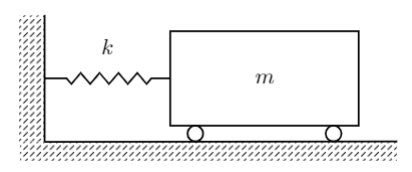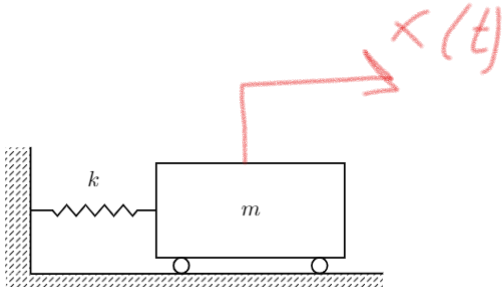In :
# Newtown's second law

In :
m,a,F, F_k,F_c,k,c,F0=symbols('m_12,a,F, F_k,F_c,k,c,F_0')

Eq(m*a,F)


$\displaystyle a m_{12} = F$
In :
# Hooke's Law - dependency which describes force of the connetor, where x is distance(displacement, exact posistion of mass place in the space, coordinate system), k is coeficient of stiffness

In :
# For the spring
eq_hook=Eq(F_k,k*x(t))
eq_hook

$\displaystyle F_{k} = k x{\left(t \right)}$
In :
# formula for acceleration

Eq(a,x(t).diff(t,2))

$\displaystyle a = \frac{d^{2}}{d t^{2}} x{\left(t \right)}$
In :
eom=Eq(m*x(t).diff(t,2),-x(t)*k )
eom

$\displaystyle m_{12} \frac{d^{2}}{d t^{2}} x{\left(t \right)} = - k x{\left(t \right)}$
In :
Eq(eom.rewrite(Add),0)

$\displaystyle k x{\left(t \right)} + m_{12} \frac{d^{2}}{d t^{2}} x{\left(t \right)} = 0$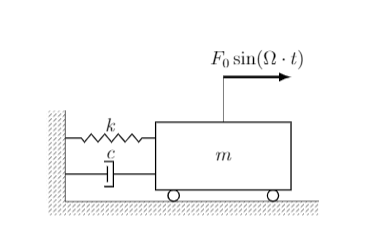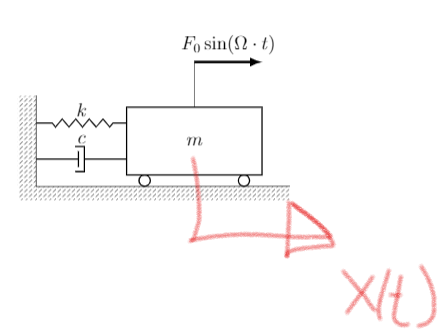In :
Omg=Symbol('\Omega')

eom=Eq(m*x(t).diff(t,2),-x(t)*k-c*x(t).diff(t) +F0*sin(Omg*t))
eom

$\displaystyle m_{12} \frac{d^{2}}{d t^{2}} x{\left(t \right)} = F_{0} \sin{\left(\Omega t \right)} - c \frac{d}{d t} x{\left(t \right)} - k x{\left(t \right)}$
In [ ]:


In :
# Describe the force of disconector, dumper, (C-element), c - dissipative property and this formula describes velocity with respect to c, dissipative(drag, ressistant) force -
# force which acts in opposite direction with respect to the main force.
# If the velocity is higher the higher drag(dumper) force
# spring tries to keep system equivalent,
# dumper just transfers the energy
Eq(F_c,c*x(t).diff(t))

$\displaystyle F_{c} = c \frac{d}{d t} x{\left(t \right)}$

https://en.wikipedia.org/wiki/Hooke's_lawIn :
# As x might represent the distance, to find the speed we need just to find derivative, Formula for kinetic energy, labor
T=S.One/2 * m * x(t).diff(t)**2
V= Integral(k*x(t),x(t))

T

$\displaystyle \frac{m_{12} \left(\frac{d}{d t} x{\left(t \right)}\right)^{2}}{2}$
In :
# https://en.wikipedia.org/wiki/Work_(physics)
#formula for potential energy

V.doit()

$\displaystyle \frac{k x^{2}{\left(t \right)}}{2}$
In :
# wrong approach - just for explanation

W=F_k *x(t)
#application of Hooke's law
W.subs(eq_hook.lhs,eq_hook.rhs)

$\displaystyle k x^{2}{\left(t \right)}$
In [ ]:
# amount of motion is kinetic energy, capacity of energy is the potential energy.

In [ ]:


In :
v=x(t).diff(t)

V=S.One/2*k* x(t)**2 + S.One/2*k*(-1*sin(Omg*t)+x(t))**2

#Solution using the lagrangian mechanics application, difference between kinetic energy and potential energy solution for the out with the first picture(out 8), picture with single degree
L=(T-V).doit()
#dissipative potential
D = S.One /2 * c  * v**2

Eq(L.diff(v).diff(t)-L.diff(x(t)) + D.diff(v),0).expand().doit()

$\displaystyle c \frac{d}{d t} x{\left(t \right)} + 2 k x{\left(t \right)} - k \sin{\left(\Omega t \right)} + m_{12} \frac{d^{2}}{d t^{2}} x{\left(t \right)} = 0$
In :
eom

$\displaystyle m_{12} \frac{d^{2}}{d t^{2}} x{\left(t \right)} = F_{0} \sin{\left(\Omega t \right)} - c \frac{d}{d t} x{\left(t \right)} - k x{\left(t \right)}$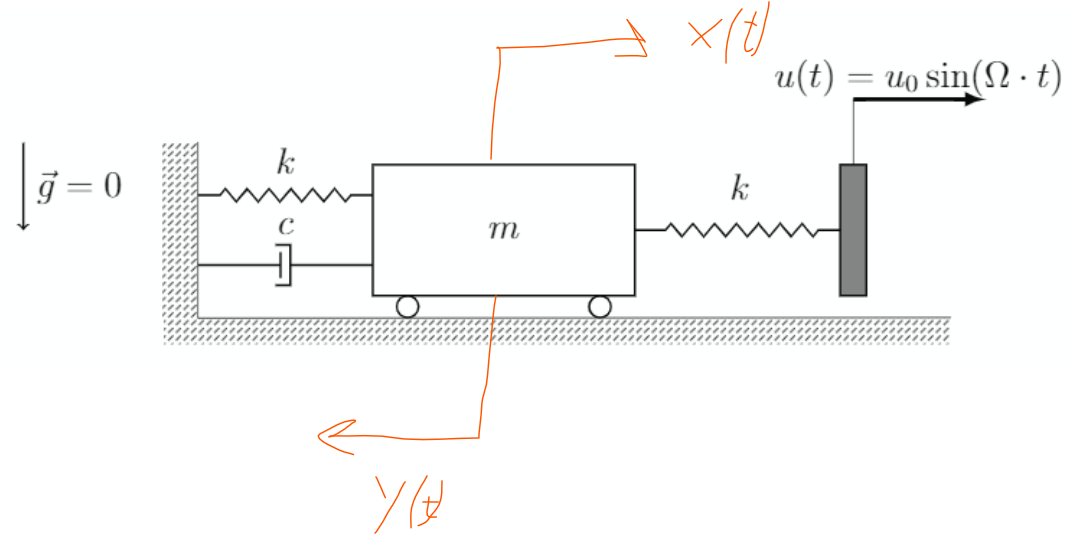In :
# in this case u is the displacement, in general it is given by the data, and aslo it can be understood from the case for the letter

eom_u0=Eq(m*x(t).diff(t,2),-x(t)*k-c*x(t).diff(t) +  k*(1*sin(Omg*t)-x(t)) )
eom_u0.expand()


$\displaystyle m_{12} \frac{d^{2}}{d t^{2}} x{\left(t \right)} = - c \frac{d}{d t} x{\left(t \right)} - 2 k x{\left(t \right)} + k \sin{\left(\Omega t \right)}$
In [ ]: